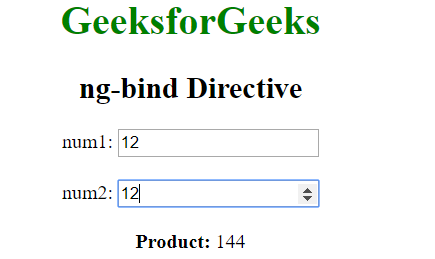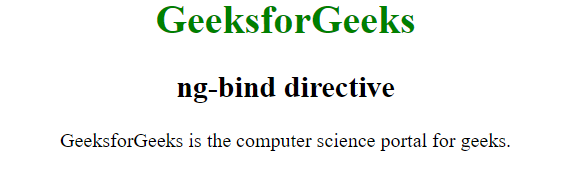# AngularJS | ng-bind Directive

The ng-bind Directive in AngularJS is used to bind/replace the text content of any particular HTML element with the value that is entered in the given expression. The value of specified HTML content updates whenever the value of the expression changes in ng-bind directive.

Syntax:

```<element ng-bind="expression"> Contents... </element>
```

Where expression is used to specify the expression to be evaluated or the variable.

Example 1: This example uses ng-bind Directive to bind the product of two number to the <span> element.

 ` ` `<``html``> ` `     `  `<``head``> ` `    ``<``title``>ng-checked Directive ` ` `  `    ``<``script` `src``= ` `"https://ajax.googleapis.com/ajax/libs/angularjs/1.6.9/angular.min.js"``> ` `    `` ` ` ` ` `  `<``body` `ng-app``=``"gfg"` `style``=``"text-align:center"``> ` `     `  `    ``<``h1` `style``=``"color:green"``>GeeksforGeeks ` `    ``<``h2``>ng-bind Directive         ` `     `  `    ``<``div` `ng-controller``=``"app"``> ` `        ``num1: <``input` `type``=``"number"` `ng-model``=``"num1"` `                ``ng-change``=``"product()"` `/> ` `        ``<``br``><``br``> ` `         `  `        ``num2: <``input` `type``=``"number"` `ng-model``=``"num2"` `                ``ng-change``=``"product()"` `/> ` `        ``<``br``><``br``> ` `         `  `        ``<``b``>Product: <``span` `ng-bind``=``"result"``> ` `    `` ` `     `  `    ``<``script``> ` `        ``var app = angular.module("gfg", []); ` `        ``app.controller('app', ['\$scope', function (\$app) { ` `            ``\$app.num1 = 1; ` `            ``\$app.num2 = 1; ` `            ``\$app.product = function () { ` `                ``\$app.result = (\$app.num1 * \$app.num2); ` `            ``} ` `        ``}]); ` `    `` ` ` ` ` `  ` `

Output:Example 2: This example uses ng-bind Directive to bind the innerHTML of the <span> element to the variable text.

 ` ` `<``html``> ` `     `  `<``head``> ` `    ``<``title``>ng-checked Directive ` ` `  `    ``<``script` `src``= ` `"https://ajax.googleapis.com/ajax/libs/angularjs/1.6.9/angular.min.js"``> ` `    `` ` ` ` ` `  `<``body`  `style` `= ``"text-align:center"``> ` `     `  `    ``<``h1` `style` `= ``"color:green"``>GeeksforGeeks ` `    ``<``h2` `style` `= ``""``>ng-bind directive ` `     `  `    ``<``div` `ng-app``=``""` `ng-init``=``"txt='GeeksforGeeks';col='green'"``> ` `        ``<``div``> ` `            ``<``span` `ng-bind``=``"txt"``> is the ` `            ``computer science portal for geeks. ` `        `` ` `    `` ` ` ` ` `  ` `

Output:My Personal Notes arrow_drop_upIf you like GeeksforGeeks and would like to contribute, you can also write an article using contribute.geeksforgeeks.org or mail your article to contribute@geeksforgeeks.org. See your article appearing on the GeeksforGeeks main page and help other Geeks.

Please Improve this article if you find anything incorrect by clicking on the "Improve Article" button below.